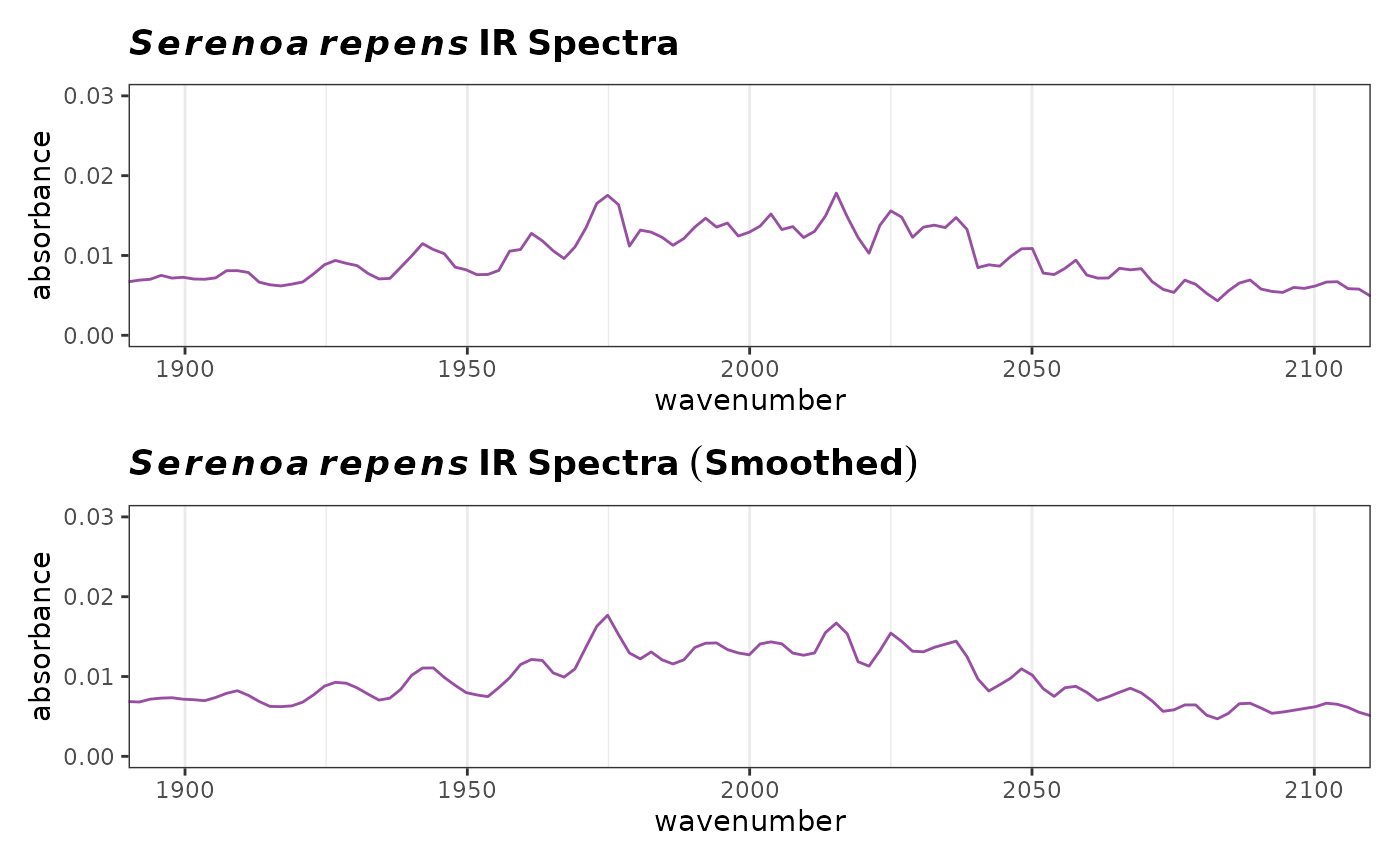This function is a simple wrapper around the function sgolayfilt. It allows one to apply Savitzky-Golay filters to a Spectra object in a convenient way.

sgfSpectra(spectra, m = 0, ...)

## Arguments

spectra

An object of S3 class Spectra to be checked.

m

The desired m-th derivative. m = 0 smooths the data (i.ei a rolling average), m = 1 gives the first derivative etc.

...

Other parameters to be passed to sgolayfilt.

## Value

A object of class Spectra.

## Author

Bryan A. Hanson (DePauw University)

## Examples


# This example assumes the graphics output is set to ggplot2 (see ?GraphicsOptions).
library("ggplot2")
library("patchwork")
data(SrE.IR)
myt1 <- expression(bolditalic(Serenoa) ~ bolditalic(repens) ~ bold(IR ~ Spectra))
myt2 <- expression(bolditalic(Serenoa) ~ bolditalic(repens) ~ bold(IR ~ Spectra ~ (Smoothed)))

p1 <- plotSpectra(SrE.IR)
p1 <- p1 + ggtitle(myt1) + coord_cartesian(xlim = c(1900, 2100), ylim = c(0.0, 0.03))
#> Coordinate system already present. Adding new coordinate system, which will replace the existing one.

sgf <- sgfSpectra(SrE.IR)
p2 <- plotSpectra(sgf)
p2 <- p2 + ggtitle(myt2) + coord_cartesian(xlim = c(1900, 2100), ylim = c(0.0, 0.03))
#> Coordinate system already present. Adding new coordinate system, which will replace the existing one.

p3 <- p1/p2
p3